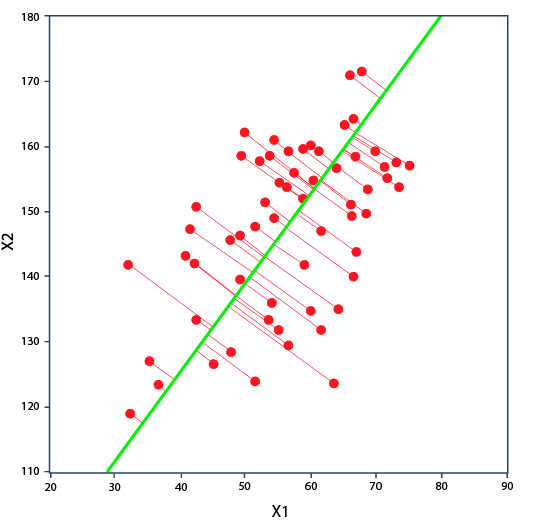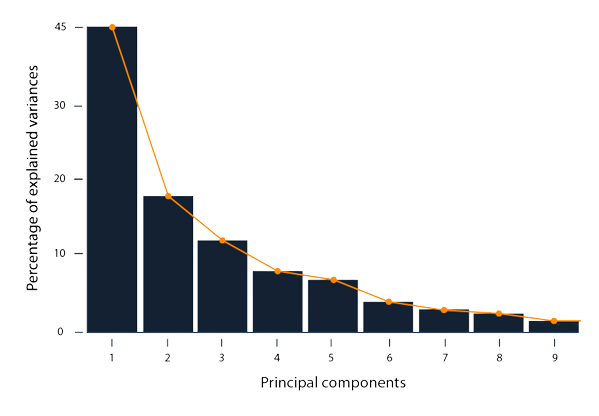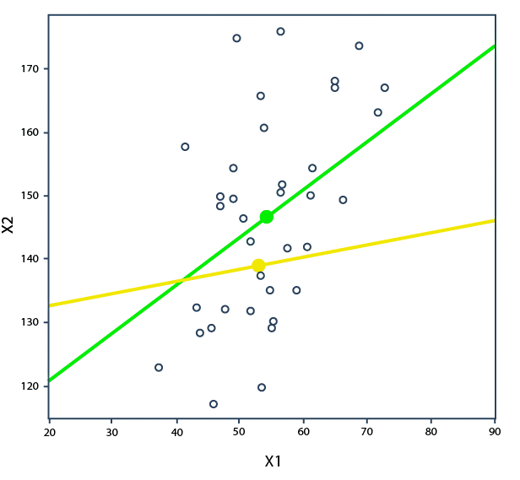Course Content

Principal Component AnalysisMathematical Idea

PCA effectively solves the problem of data compression with the least loss. Let's try to imagine how this happens mathematically.

If we have images that we want to "compress", it is logical to leave the most important and pronounced parts of the image, and the details can be removed.

The PCA algorithm works the same way. It combines the most correlated variables into new variables (components). But here is an important detail! Components among themselves should have the least correlation for the severity of the data.

Mathematically, this problem can be referred to as the problem of maximizing the variance between data points. Thus, the main components are formed, which the PCA algorithm creates.The first component "holds" the largest amount of data, while the second, third and subsequent - less and less.In the second section, we will take a closer look at each step on the way to deriving the principal components.

## Quiz

We decided to use PCA to convert data from 2D to 1D space.